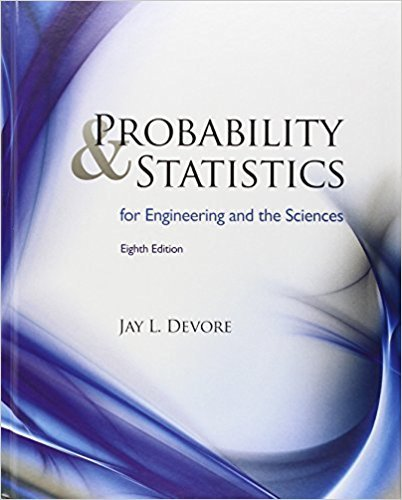×
×

# Solutions for Chapter 8: Tests of Hypotheses Based on a Single Sample## Full solutions for Probability and Statistics for Engineering and the Sciences | 8th Edition

ISBN: 9780538733526Solutions for Chapter 8: Tests of Hypotheses Based on a Single Sample

Solutions for Chapter 8
4 5 0 342 Reviews
15
4
##### ISBN: 9780538733526

Probability and Statistics for Engineering and the Sciences was written by and is associated to the ISBN: 9780538733526. This textbook survival guide was created for the textbook: Probability and Statistics for Engineering and the Sciences , edition: 8. Since 87 problems in chapter 8: Tests of Hypotheses Based on a Single Sample have been answered, more than 25255 students have viewed full step-by-step solutions from this chapter. This expansive textbook survival guide covers the following chapters and their solutions. Chapter 8: Tests of Hypotheses Based on a Single Sample includes 87 full step-by-step solutions.

Key Statistics Terms and definitions covered in this textbook
• Bias

An effect that systematically distorts a statistical result or estimate, preventing it from representing the true quantity of interest.

• C chart

An attribute control chart that plots the total number of defects per unit in a subgroup. Similar to a defects-per-unit or U chart.

• Central composite design (CCD)

A second-order response surface design in k variables consisting of a two-level factorial, 2k axial runs, and one or more center points. The two-level factorial portion of a CCD can be a fractional factorial design when k is large. The CCD is the most widely used design for itting a second-order model.

• Conditional probability mass function

The probability mass function of the conditional probability distribution of a discrete random variable.

• Conidence interval

If it is possible to write a probability statement of the form PL U ( ) ? ? ? ? = ?1 where L and U are functions of only the sample data and ? is a parameter, then the interval between L and U is called a conidence interval (or a 100 1( )% ? ? conidence interval). The interpretation is that a statement that the parameter ? lies in this interval will be true 100 1( )% ? ? of the times that such a statement is made

• Contingency table.

A tabular arrangement expressing the assignment of members of a data set according to two or more categories or classiication criteria

• Continuity correction.

A correction factor used to improve the approximation to binomial probabilities from a normal distribution.

• Covariance

A measure of association between two random variables obtained as the expected value of the product of the two random variables around their means; that is, Cov(X Y, ) [( )( )] =? ? E X Y ? ? X Y .

• Critical region

In hypothesis testing, this is the portion of the sample space of a test statistic that will lead to rejection of the null hypothesis.

• Deming’s 14 points.

A management philosophy promoted by W. Edwards Deming that emphasizes the importance of change and quality

• Dependent variable

The response variable in regression or a designed experiment.

• Discrete distribution

A probability distribution for a discrete random variable

• Discrete random variable

A random variable with a inite (or countably ininite) range.

• Discrete uniform random variable

A discrete random variable with a inite range and constant probability mass function.

• Error sum of squares

In analysis of variance, this is the portion of total variability that is due to the random component in the data. It is usually based on replication of observations at certain treatment combinations in the experiment. It is sometimes called the residual sum of squares, although this is really a better term to use only when the sum of squares is based on the remnants of a model-itting process and not on replication.

• Experiment

A series of tests in which changes are made to the system under study

• Factorial experiment

A type of experimental design in which every level of one factor is tested in combination with every level of another factor. In general, in a factorial experiment, all possible combinations of factor levels are tested.

• Finite population correction factor

A term in the formula for the variance of a hypergeometric random variable.

• Fractional factorial experiment

A type of factorial experiment in which not all possible treatment combinations are run. This is usually done to reduce the size of an experiment with several factors.

• Frequency distribution

An arrangement of the frequencies of observations in a sample or population according to the values that the observations take on

×